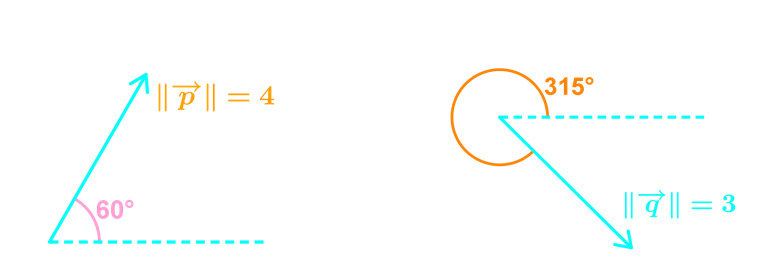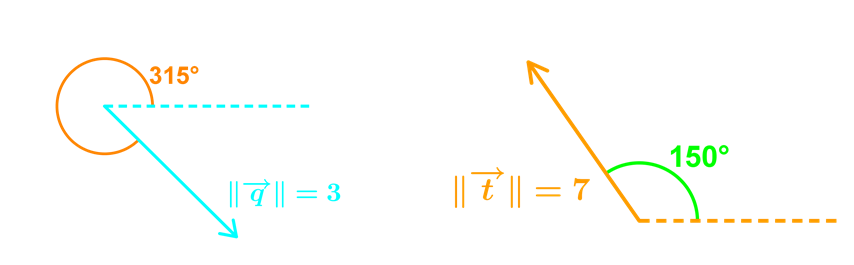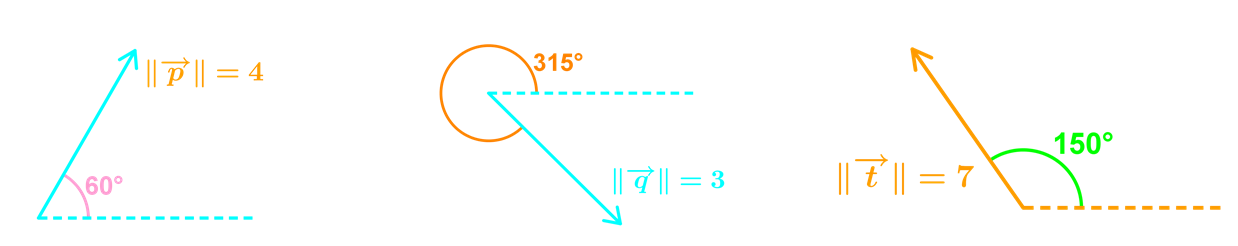# Operations on vectors in magnitude and direction form#### Everything You Need in One Place

Homework problems? Exam preparation? Trying to grasp a concept or just brushing up the basics? Our extensive help & practice library have got you covered.#### Learn and Practice With Ease

Our proven video lessons ease you through problems quickly, and you get tonnes of friendly practice on questions that trip students up on tests and finals.#### Instant and Unlimited Help

Our personalized learning platform enables you to instantly find the exact walkthrough to your specific type of question. Activate unlimited help now!##### Examples
###### Lessons
1. Given the following vectors for $\vec{p}$, $\vec{q}$, $\vec{t}$find $\vec{p}+\vec{q}$
1. Given the following vectors for $\vec{p}$, $\vec{q}$, $\vec{t}$find $\vec{t}+\vec{p}$
1. Given the following vectors for $\vec{p}$, $\vec{q}$, $\vec{t}$find $\vec{p}+\vec{q}+\vec{t}$
1. Particle $\beta$ is acted upon by the following forces:

• Force A with a magnitude of 30N towards north west
• Force B with a magnitude of 20N towards East
• Force C with a magnitude of 15N in the direction of 25° south of west
1. find the magnitude of the resultant force
2. find the direction of the resultant force
###### Topic Notes
By now we should be able to add or subtract vectors if we are given their components, but what if we are only given their magnitudes and direction angles instead? In this section, we will learn how to perform operations on vectors presented in magnitude and direction form, using the "three-steps approach".